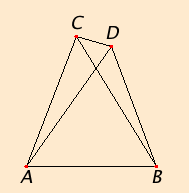# Proposition 7

Given two straight lines constructed from the ends of a straight line and meeting in a point, there cannot be constructed from the ends of the same straight line, and on the same side of it, two other straight lines meeting in another point and equal to the former two respectively, namely each equal to that from the same end.

If possible, given two straight lines AC and CB constructed on the straight line AB and meeting at the point C, let two other straight lines AD and DB be constructed on the same straight line AB, on the same side of it, meeting in another point D and equal to the former two respectively, namely each equal to that from the same end, so that AC equals AD which has the same end A, and CB equals DB which has the same end B.I.Post.1

Join CD.

Since AC equals AD, therefore the angle ACD equals the angle ADC. Therefore the angle ADC is greater than the angle DCB. Therefore the angle CDB is much greater than the angle DCB.

Again, since CB equals DB, therefore the angle CDB also equals the angle DCB. But it was also proved much greater than it, which is impossible.

Therefore given two straight lines constructed from the ends of a straight line and meeting in a point, there cannot be constructed from the ends of the same straight line, and on the same side of it, two other straight lines meeting in another point and equal to the former two respectively, namely each equal to that from the same end.

Q.E.D.

## Guide

In order to conclude “the angle ADC is greater than the angle DCB” it is necessary for angle ADC to be greater than angle DCB, but that won’t happen unless the point D lies outside the triangle ABC. Euclid hasn’t considered the case when D lies inside triangle ABC as well as other special cases. This is not unusual as Euclid frequently treats only one case. Commentators over the centuries have inserted other cases in this and other propositions. It is usually easy to modify Euclid’s proof for the remaining cases. In this proposition for the case when D lies inside triangle ABC, the second conclusion of I.5 may be used to justify the proof.

#### Hidden justifications

The sentences
... the angle ACD equals the angle ADC. Therefore the angle ADC is greater than the angle DCB. Therefore the angle CDB is much greater than the angle DCB.
use several properties of magnitudes. C.N.5 justifies the unstated angle ACD > DCB since DCB is part of ACD. The statement that ADC is greater than the angle DCB is justified by the property of magnitudes

If x < y and y = z, then x < z.

This property is not among the listed Common Notions.

Next, transitivity of “less than”

If x < y and y < z, then x < z.

justifies the last statement “CDB is much greater than the angle DCB.” Transitivity is another property not listed as a Common Notion.

As in the proof of the last proposition and many to come, the law of trichotomy is also used. Here it’s used to reach the final contradiction.

#### Use of Proposition 7

This proposition is used in the proof of the next proposition.